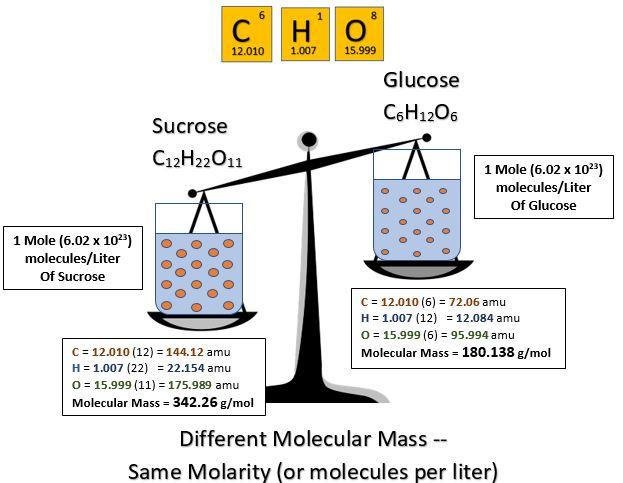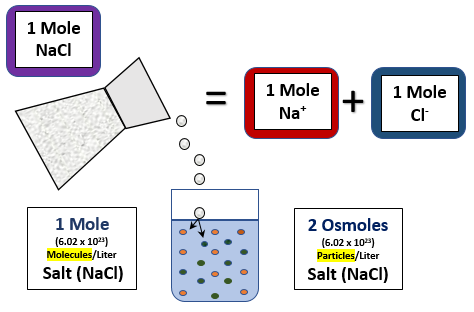2.2

# Water and Aqueous Solutions

As mentioned above, before chemicals can react in chemical reactions, they must be in solution. As it turns out, water is an excellent solvent. A solvent is a dissolving agent and is the liquid portion of a solution. The molecules that dissolve in the solvent are called the solutes. Therefore, in a solution of salt (NaCl) and water, the water is the solvent, and the sodium and chloride are the solutes. A solution in which water is the solvent is called an aqueous solution. Although water is an excellent solvent, not everything dissolves readily in water. Materials that dissolve well in water are said to be hydrophilic (hydro- = water; -phil- = love), and those that do not dissolve readily are said to be hydrophobic (phobia = fear). Usually, if we know the chemical nature of a solute, we can predict how readily it will dissolve in water. For example, compounds that are bound together by ionic bonds tend to be hydrophilic and dissolve readily. The secret is the ability of the polar water molecules to surround the ions and pull them out of the crystal. When the ions are pulled apart in this manner, we say the compound has become dissociated or ionized. The dissociated ions in the solution are referred to as electrolytes. Important electrolytes for life include Na+, K+, Ca2+, Cl-, HCO3-, H+, and Mg2+. These ions participate in many important physiological processes such as nerve impulse conduction, muscle contraction, and regulating water balance.

In addition to ionic compounds, compounds bound together with polar covalent bonds also tend to be hydrophilic. Sucrose, or table sugar (C12H22O11), is a good example of a polar compound that readily dissolves in water, forming an aqueous solution. However, when polar covalent molecules dissolve in water, they do not ionize or separate into smaller particles like ionic compounds do.

Compounds bound together with nonpolar covalent bonds tend to be hydrophobic and do not dissolve readily in water. This is because there are no charged or polar parts to interact with the polar water molecules. Fats and oils are good examples of compounds that are hydrophobic.

One of the most important structures in cells is the biological membrane. These membranes are stabilized by the hydrophobic and hydrophilic interactions of some special compounds that we will study later.

#### Solute Concentration

It is often important to know the concentration of the solute in a solution. Some common ways of expressing concentration are straightforward. For example, the normal fasting glucose concentration in human blood is approximately 90 mg glucose per 100 ml of blood. This would be written as 90 mg/dl (dl stands for deciliter or 1/10 of a liter). Another fairly simple method is to express the concentration of the solute in a percent solution. This method expresses the concentration as grams of solute per 100 ml of solvent. For example, the normal concentration of NaCl in human blood is 0.9%. This means that there is 0.9 g of NaCl per 100 ml of blood plasma. Both of these methods are fairly easy to visualize. However, they are not very precise. A less obvious but more precise method is to express the concentration as the molarity of the solution. Let’s see if we can walk through what this means. First of all, the term molarity means moles of solute per liter of solution. So, the next question is, what the heck is a mole?

To begin, a mole is a unit of measurement. In order to understand what is meant by “unit of measurement,” let’s start with something you are familiar with, the dozen. We are tempted to ask, “Where in the world did that word come from, and why does it signify the value of 12?” The value of 12 is a unique number because of the early observations of the cycles of the moon, which led to the proposed twelve-month cycle of a year. After a gradual shorting of the Latin word for twelve, “duodecim”, the English derivation of this word became the word dozen—a grouped quantity, signifying the value of twelve. Why group things? For starters, it is easier to go to the store and buy 12 dozen eggs than it is to individually count out 144 eggs. Units of measurements allow us to conveniently talk about large numbers at an understandable level. The concept of unit measurement is absolutely essential when it comes to counting atoms.

Since it is nearly impossible for us to measure 12 atoms, we need to use an understandable unit of measurement.  A universally recognized unit of measurement for atoms (or molecules) is the mole (abbreviated mol). Just like one dozen is equivalent to 12, it has been determined that one mole is equivalent to 6.02 X 1023 atoms. The number is called Avogadro's number and is named after the famous scientist, Amedeo Avogadro (1776-1856). Just how big is a mole? If we were to go to a store and buy a bunch of eggs equivalent to the number of molecules in a mole, we could fill the volume of the earth approximately 40 times! Thankfully, molecules are much smaller than eggs; in fact, a mole of sucrose (table sugar) weighs only 342 grams and would barely fill the volume of a tennis ball. The difference, of course, is the size of the atoms that make up the sucrose molecule. So, how did we determine that 342 grams of sucrose contain Avogadro’s number of molecules? First, we need to determine the molecular mass of sucrose. To do this, we take the atomic mass of each atom in the compound and add them together. For example, the formula for sucrose is C12H22O11. The atomic mass of carbon is 12, and there are 12 carbon atoms in sucrose, so 12 x 12 = 144. The atomic mass of hydrogen is 1, and there are 22 hydrogen atoms in sucrose, so 22 x 1 = 22. Finally, the atomic mass of oxygen is 16, and there are 11 oxygen atoms in sucrose, so 11 x 16 = 176. When we add these together, we get 144 + 22 + 176 = 342. Therefore, the molecular mass of sucrose is 342 (we have rounded the atomic masses to the nearest whole number to make the computations easier). The units for this weight are atomic mass units (amu). Therefore, one sucrose molecule weighs 342 amu and one mole of sucruose weighs 342 grams.

This is much too small of a mass to weigh out on any existing scale (recall that one amu is approximately the mass of a proton or a neutron). However, we can easily weight out 342 g of sucrose. The amu total for a molecule expressed in grams is equal to one mole of that molecule. If we take that amount of sucrose (342 g) and add water until we have one liter of solution, we will have a one molar solution of sucrose. So, in a one molar solution of sucrose, we have 342 g, one mole, or 6.02 x 1023 molecules in one liter of solution. The advantage of expressing concentrations in molarity is that it is an expression that lets us compare the number of molecules in the solutions. For example, a one molar solution of sucrose (molecular mass = 342) and a one molar solution of glucose (molecular mass = 180) will have exactly the same number of molecules per liter of solution even though the molecules are different in size, and we may have added different masses of each.One other expression of concentration that is often useful is Osmolarity, which, as you can probably guess, is Osmoles per liter. This is a lot like molarity except that molarity is 6.02 x 1023 molecules per liter, whereas osmolarity is 6.02 x 1023 particles per liter. At first, it may seem like molecules and particles are the same thing. However, if a solute dissociates (comes apart) when dissolved, you end up with more than one particle per molecule. Take NaCl (salt) for example. When you dissolve one mole of salt in water, each molecule splits in two, so you end up with 2 x (6.02 x 1023 particles) in the solution. Hence, a one molar NaCl solution would be a two osmolar NaCl solution (NaCl is a molecule, and Na+ and Cl+ are particles). *Note: the actual osmolar NaCl solution is 1.6 because complete dissociation doesn’t actually occur, but this will take on more significance when we talk about osmosis later in the course.